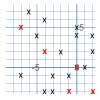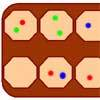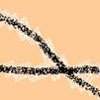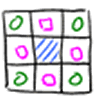# Resources tagged with: Visualising

Filter by: Content type:
Age range:
Challenge level:

### There are 179 results

Broad Topics > Thinking Mathematically > Visualising### A Tilted Square

##### Age 14 to 16 Challenge Level:

The opposite vertices of a square have coordinates (a,b) and (c,d). What are the coordinates of the other vertices?### Square Coordinates

##### Age 11 to 14 Challenge Level:

A tilted square is a square with no horizontal sides. Can you devise a general instruction for the construction of a square when you are given just one of its sides?### Dissect

##### Age 11 to 14 Challenge Level:

What is the minimum number of squares a 13 by 13 square can be dissected into?### Chess

##### Age 11 to 14 Challenge Level:

What would be the smallest number of moves needed to move a Knight from a chess set from one corner to the opposite corner of a 99 by 99 square board?### Ten Hidden Squares

##### Age 7 to 14 Challenge Level:

These points all mark the vertices (corners) of ten hidden squares. Can you find the 10 hidden squares?### Wari

##### Age 14 to 16 Challenge Level:

This is a simple version of an ancient game played all over the world. It is also called Mancala. What tactics will increase your chances of winning?### Coloured Edges

##### Age 11 to 14 Challenge Level:

The whole set of tiles is used to make a square. This has a green and blue border. There are no green or blue tiles anywhere in the square except on this border. How many tiles are there in the set?### Coordinate Patterns

##### Age 11 to 14 Challenge Level:

Charlie and Alison have been drawing patterns on coordinate grids. Can you picture where the patterns lead?##### Age 11 to 14 Challenge Level:

Can you mark 4 points on a flat surface so that there are only two different distances between them?### Square It

##### Age 11 to 16 Challenge Level:

Players take it in turns to choose a dot on the grid. The winner is the first to have four dots that can be joined to form a square.### Eight Hidden Squares

##### Age 7 to 14 Challenge Level:

On the graph there are 28 marked points. These points all mark the vertices (corners) of eight hidden squares. Can you find the eight hidden squares?### Something in Common

##### Age 14 to 16 Challenge Level:

A square of area 3 square units cannot be drawn on a 2D grid so that each of its vertices have integer coordinates, but can it be drawn on a 3D grid? Investigate squares that can be drawn.### Screwed-up

##### Age 11 to 14 Challenge Level:

A cylindrical helix is just a spiral on a cylinder, like an ordinary spring or the thread on a bolt. If I turn a left-handed helix over (top to bottom) does it become a right handed helix?### Corridors

##### Age 14 to 16 Challenge Level:

A 10x10x10 cube is made from 27 2x2 cubes with corridors between them. Find the shortest route from one corner to the opposite corner.### Concrete Wheel

##### Age 11 to 14 Challenge Level:

A huge wheel is rolling past your window. What do you see?### Making Tracks

##### Age 14 to 16 Challenge Level:

A bicycle passes along a path and leaves some tracks. Is it possible to say which track was made by the front wheel and which by the back wheel?### Just Opposite

##### Age 14 to 16 Challenge Level:

A and C are the opposite vertices of a square ABCD, and have coordinates (a,b) and (c,d), respectively. What are the coordinates of the vertices B and D? What is the area of the square?### 3D Stacks

##### Age 7 to 14 Challenge Level:

Can you find a way of representing these arrangements of balls?### Route to Infinity

##### Age 11 to 14 Challenge Level:

Can you describe this route to infinity? Where will the arrows take you next?### Thinking Through, and By, Visualising

##### Age 7 to 16

This article is based on some of the ideas that emerged during the production of a book which takes visualising as its focus. We began to identify problems which helped us to take a structured view. . . .### Rati-o

##### Age 11 to 14 Challenge Level:

Points P, Q, R and S each divide the sides AB, BC, CD and DA respectively in the ratio of 2 : 1. Join the points. What is the area of the parallelogram PQRS in relation to the original rectangle?### Dotty Triangles

##### Age 11 to 14 Challenge Level:

Imagine an infinitely large sheet of square dotty paper on which you can draw triangles of any size you wish (providing each vertex is on a dot). What areas is it/is it not possible to draw?### Tilting Triangles

##### Age 14 to 16 Challenge Level:

A right-angled isosceles triangle is rotated about the centre point of a square. What can you say about the area of the part of the square covered by the triangle as it rotates?### Hidden Rectangles

##### Age 11 to 14 Challenge Level:

Rectangles are considered different if they vary in size or have different locations. How many different rectangles can be drawn on a chessboard?### Squares, Squares and More Squares

##### Age 11 to 14 Challenge Level:

Can you dissect a square into: 4, 7, 10, 13... other squares? 6, 9, 12, 15... other squares? 8, 11, 14... other squares?### Convex Polygons

##### Age 11 to 14 Challenge Level:

Show that among the interior angles of a convex polygon there cannot be more than three acute angles.### Hypotenuse Lattice Points

##### Age 14 to 16 Challenge Level:

The triangle OMN has vertices on the axes with whole number co-ordinates. How many points with whole number coordinates are there on the hypotenuse MN?### Pattern Power

##### Age 5 to 14

Mathematics is the study of patterns. Studying pattern is an opportunity to observe, hypothesise, experiment, discover and create.### Framed

##### Age 11 to 14 Challenge Level:

Seven small rectangular pictures have one inch wide frames. The frames are removed and the pictures are fitted together like a jigsaw to make a rectangle of length 12 inches. Find the dimensions of. . . .### Zooming in on the Squares

##### Age 7 to 14

Start with a large square, join the midpoints of its sides, you'll see four right angled triangles. Remove these triangles, a second square is left. Repeat the operation. What happens?### Isosceles Triangles

##### Age 11 to 14 Challenge Level:

Draw some isosceles triangles with an area of $9$cm$^2$ and a vertex at (20,20). If all the vertices must have whole number coordinates, how many is it possible to draw?### All in the Mind

##### Age 11 to 14 Challenge Level:

Imagine you are suspending a cube from one vertex and allowing it to hang freely. What shape does the surface of the water make around the cube?### Jam

##### Age 14 to 16 Challenge Level:

To avoid losing think of another very well known game where the patterns of play are similar.### Tetra Square

##### Age 11 to 14 Challenge Level:

ABCD is a regular tetrahedron and the points P, Q, R and S are the midpoints of the edges AB, BD, CD and CA. Prove that PQRS is a square.### Inside Out

##### Age 14 to 16 Challenge Level:

There are 27 small cubes in a 3 x 3 x 3 cube, 54 faces being visible at any one time. Is it possible to reorganise these cubes so that by dipping the large cube into a pot of paint three times you. . . .##### Age 11 to 14 Challenge Level:

Four rods, two of length a and two of length b, are linked to form a kite. The linkage is moveable so that the angles change. What is the maximum area of the kite?### Triangles Within Pentagons

##### Age 14 to 16 Challenge Level:

Show that all pentagonal numbers are one third of a triangular number.### Partly Painted Cube

##### Age 14 to 16 Challenge Level:

Jo made a cube from some smaller cubes, painted some of the faces of the large cube, and then took it apart again. 45 small cubes had no paint on them at all. How many small cubes did Jo use?### Christmas Chocolates

##### Age 11 to 14 Challenge Level:

How could Penny, Tom and Matthew work out how many chocolates there are in different sized boxes?### Mystic Rose

##### Age 14 to 16 Challenge Level:

Use the animation to help you work out how many lines are needed to draw mystic roses of different sizes.### Speeding Boats

##### Age 14 to 16 Challenge Level:

Two boats travel up and down a lake. Can you picture where they will cross if you know how fast each boat is travelling?### Baravelle

##### Age 7 to 16 Challenge Level:

What can you see? What do you notice? What questions can you ask?### Cubes Within Cubes Revisited

##### Age 11 to 14 Challenge Level:

Imagine starting with one yellow cube and covering it all over with a single layer of red cubes, and then covering that cube with a layer of blue cubes. How many red and blue cubes would you need?### Triangles Within Triangles

##### Age 14 to 16 Challenge Level:

Can you find a rule which connects consecutive triangular numbers?### Weighty Problem

##### Age 11 to 14 Challenge Level:

The diagram shows a very heavy kitchen cabinet. It cannot be lifted but it can be pivoted around a corner. The task is to move it, without sliding, in a series of turns about the corners so that it. . . .### Sliding Puzzle

##### Age 11 to 16 Challenge Level:

The aim of the game is to slide the green square from the top right hand corner to the bottom left hand corner in the least number of moves.### Changing Places

##### Age 14 to 16 Challenge Level:

Place a red counter in the top left corner of a 4x4 array, which is covered by 14 other smaller counters, leaving a gap in the bottom right hand corner (HOME). What is the smallest number of moves. . . .##### Age 14 to 16 Challenge Level:

In this problem we are faced with an apparently easy area problem, but it has gone horribly wrong! What happened?### On the Edge

##### Age 11 to 14 Challenge Level:

If you move the tiles around, can you make squares with different coloured edges?### Jam

##### Age 14 to 16 Challenge Level:

A game for 2 players